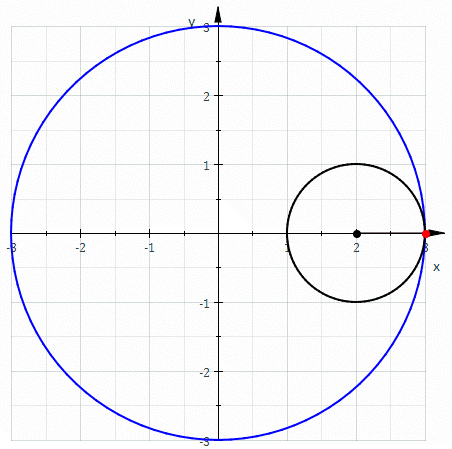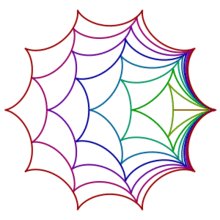# HypocycloidThe red curve is a hypocycloid traced as the smaller black circle rolls around inside the larger blue circle (parameters are R=3.0, r=1.0, and so k=3, giving a deltoid).

In geometry, a hypocycloid is a special plane curve generated by the trace of a fixed point on a small circle that rolls within a larger circle. It is comparable to the cycloid but instead of the circle rolling along a line, it rolls within a circle.

## Properties

If the smaller circle has radius r, and the larger circle has radius R = kr, then the parametric equations for the curve can be given by either:or:If k is an integer, then the curve is closed, and has k cusps (i.e., sharp corners, where the curve is not differentiable). Specially for k=2 the curve is a straight line and the circles are called Cardano circles. Girolamo Cardano was the first to describe these hypocycloids and their applications to high-speed printing.

If k is a rational number, say k = p/q expressed in simplest terms, then the curve has p cusps.

If k is an irrational number, then the curve never closes, and fills the space between the larger circle and a circle of radius R − 2r.

Each hypocycloid (for any value of r) is a brachistochrone for the gravitational potential inside a homogeneous sphere of radius R.

## Examples

The hypocycloid is a special kind of hypotrochoid, which are a particular kind of roulette.

A hypocycloid with three cusps is known as a deltoid.

A hypocycloid curve with four cusps is known as an astroid.

The hypocycloid with two cusps is a degenerate but still very interesting case, known as the Tusi couple.

## Relationship to group theoryHypocycloids "rolling" inside one another. The cusps of each of the smaller curves maintain continuous contact with the next-larger hypocycloid.

Any hypocycloid with an integral value of k, and thus k cusps, can move snugly inside another hypocycloid with k+1 cusps, such that the points of the smaller hypocycloid will always be in contact with the larger. This motion looks like 'rolling', though it is not technically rolling in the sense of classical mechanics, since it involves slipping.

Hypocycloid shapes can be related to special unitary groups, denoted SU(k), which consist of k × k unitary matrices with determinant 1. For example, the allowed values of the sum of diagonal entries for a matrix in SU(3), are precisely the points in the complex plane lying inside a hypocycloid of three cusps (a deltoid). Likewise, summing the diagonal entries of SU(4) matrices give points inside an astroid, and so on.

Thanks to this result, one can use the fact that SU(k) fits inside SU(k+1) as a subgroup to prove that an epicycloid with k cusps moves snugly inside one with k+1 cusps.

## Derived curves

The evolute of a hypocycloid is an enlarged version of the hypocycloid itself, while the involute of a hypocycloid is a reduced copy of itself.

The pedal of a hypocycloid with pole at the center of the hypocycloid is a rose curve.

The isoptic of a hypocycloid is a hypocycloid.

Curves similar to hypocyloids can be drawn with the Spirograph toy. Specifically, the Spirograph can draw hypotrochoids and epitrochoids.

The Pittsburgh Steelers' logo, which is based on the Steelmark, includes three astroids (hypocycloids of four cusps). In his weekly NFL.com column Tuesday Morning Quarterback, Gregg Easterbrook often refers to the Steelers as the Hypocycloids. Chilean soccer team CD Huachipato based their crest on the Steelers' logo, and as such features hypocycloids.

The first Drew Carey season of The Price Is Right's set features astroids on the three main doors, giant price tag, and the turntable area. The astroids on the doors and turntable were removed when the show switched to high definition broadcasts starting in 2008, and only the giant price tag prop still features them today.Wikimedia Commons has media related to Hypocycloid.